•研究论文
• 本文介绍了RC带阻滤波器的工作原理和Simulink的仿真模型，传递函数和傅里叶变换关系，介绍了离散变化和连线变化对结果差异的分析，以及截止频率计算方法
• 运用labview软件，通过参数设置，实现数字带通、带阻滤波器的设计。并通过对数字信号的滤波应用进行特性分析，与理论验证。
• 通过全波分析软件Ansoft Ensemble模拟分析带阻滤波器的特性，用试验验证了模拟分析的结果。本文还应用电磁阻带结构设计了一个双工器，它采用2个电磁阻带滤波器和1个T分支级联形成三端口元件，实验表明其具有优良的...
• [例4-4] 试设计一个满足下列技术指标的BW型模拟带阻滤波器. wp1 = 6 rad/s, wp2 = 13 rad/s, ws1 = 9rad/s, ws2 = 11 rad/s, Ap <= 1dB, As >= 10dB. [问题分析] (1)根据带阻滤波器的阻带上下截频, 确定...
试设计一个满足下列技术指标的BW型模拟带阻滤波器.

wp1 = 6 rad/s, wp2 = 13 rad/s, ws1 = 9rad/s, ws2 = 11 rad/s, Ap <= 1dB, As >= 10dB.

[设计思路分析]

(1)根据带阻滤波器的阻带上下截频, 确定带阻滤波器的阻带宽度B和带阻滤波器的中心频率w0,

其中 B=ws2-ws1, wo^2=ws2·ws1;

(2)确定原型低通滤波器的通带截频wp'、阻带截频ws':

wp1' = (B·wp1) / (-wp1^2 + w0^2);

wp2' = (B·wp2) / (-wp2^2 + wo^2);

wp' = max{ |wp1'|, |wp2'| };

ws' = 1.

(3)设计技术指标为wp'、ws', Ap <= 1dB, As >= 10dB 的BW型模拟低通滤波器HL(s'):

由BW型模拟低通滤波器关于N的不等式可计算出N的值, 进而可计算出wc'的值.

这里经过较为复杂的计算, 可得出待设计模拟低通滤波器的系统函数HL(s').

(4)由复频率转换将原型低通滤波器HL(s')转换为带阻滤波器HBS(s).

[设计代码]

% 设计BW型模拟带阻滤波器
% 技术指标
wp1 = 6; wp2 = 13; ws1 = 9; ws2 = 11; Ap = 1; As = 10;
% (1)根据带阻滤波器的阻带上下截频, 确定带阻滤波器的阻带宽度B和带阻滤波器的中心频率w0
B = ws2 - ws1;
w0 = sqrt(ws2 * ws1);
% (2)确定原型低通滤波器的通带截频wp'、阻带截频ws'
wp1_ = (B * wp1) / (- wp1 * wp1 + w0 * w0);
wp2_ = (B * wp2) / (- wp2 * wp2 + w0 * w0);
if abs(wp1_) > abs(wp2_)
wp_ = abs(wp1_);
else
wp_ = abs(wp2_);
end
% 原型低通滤波器阻带截频取1
ws_ = 1;
% (3)设计技术指标为wp'、ws', Ap <= 1dB, As >= 10dB 的BW型模拟低通滤波器HL(s')
[N ,wc_] = buttord(wp_, ws_, Ap, As, 's');
[num, den] = butter(N, wc_, 's');
% (4)由复频率转换将原型低通滤波器HL(s')转换为带阻滤波器HBS(s)
[numt, dent] = lp2bs(num, den, w0, B);
% (5)画出带阻滤波器的增益响应
% 在[0, 20]区间上设置个3000个采样点
w = linspace(0, 20, 3000);
% freqs()返回一个模拟滤波器H(jw)的复频域响应(Laplace格式)
% freqs(num, den, w) -> 根据系数向量num, den计算并返回模拟滤波器的复频域响应H(s)
% 角频率w确定了输入的实向量, 因此必须包含至少一个频率点
h = freqs(numt, dent, w);
% 绘制所设计带阻滤波器的增益响应曲线
plot(w, 20 * log10(abs(h)));
% 输出所设计滤波器的重要参考指标
f = [wp1, ws1, ws2, wp2];
h = freqs(numt, dent, f);
fprintf('Ap1 = %.4f\n', -20 * log10(abs(h(1))));
fprintf('As1 = %.4f\n', -20 * log10(abs(h(2))));
fprintf('As2 = %.4f\n', -20 * log10(abs(h(3))));
fprintf('Ap2 = %.4f\n', -20 * log10(abs(h(4))));

[运行结果]

由实际仿真结果可知, Ap1 = 0.0511dB <= 1dB, As1 = 10dB, As2 = 10dB, Ap2 = 0.6867dB, 由此可见所设计模拟带阻滤波器满足技术指标.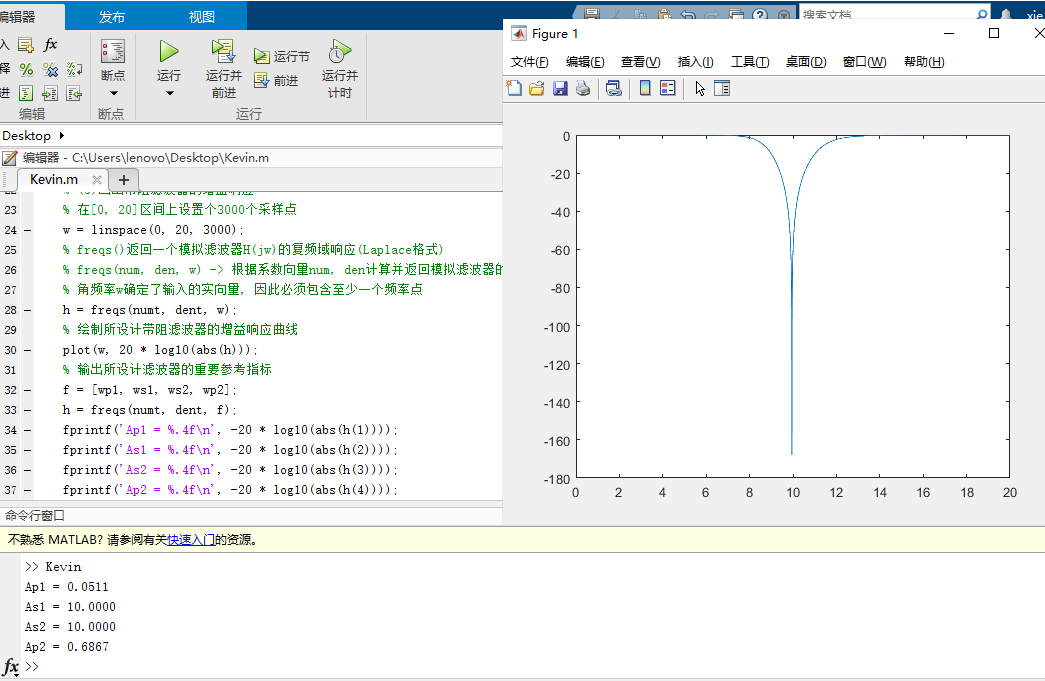展开全文• 提出了基于对偶复合左右手(Double Composite Right/Left-Handed Cell,DCRLH)单元的小型化带阻滤波器设计方案。通过对DCRLH单元等效电路模型的理论分析证明该结构在平衡状态下左右手通带无法合并,具有天然的带阻特性...
• 提出了一种基于共面波导的螺旋型缺陷地结构,利用三维电磁场仿真软件分析了该缺陷地...根据所提取的电路参数设计了一个带阻滤波器。该带阻滤波器由三个螺旋型缺陷地结构单元级联构成,仿真结果表明其具有良好的带阻特性。
• 通过将两个耦合谐振器对与馈线耦合在一起，可以实现新颖的二阶带阻滤波器，并将其与天线集成在一起以抑制上下WLAN频段。 通过实验对陷波带性能进行了分析和验证。 仿真和测量结果表明，所提出的天线能够成功地从3...
• 带阻滤波器matlab代码 频谱：用于神经时间序列分析（v1.0）的工具箱 目录 ：关于此存储库和代码 1.1：模块列表 ：文件夹的组织和结构 ：安装，执行 3.1 3.2 1.什么 包含用于Spectral的Matlab和Python脚本，Spectral...
• 缺陷接地结构（DGS）作为频率选择表面（FSS）的一类，在电磁领域应用广泛。...这些有益的结论能为这种新颖DGS带阻滤波器分析和设计提供有力的指导。讨论了CN-FDTD的误差项，随着时间步长的增大，其误差也会显著增大。
• 提出了一种利用双模环形谐振器实现的带阻滤波器。该滤波器由一个对称的双模环形谐振器以及一段四 分之一波长的平行耦合线组成。借助于在普通环形谐振器的两个相对边上添加一对小贴片,环形谐振器的两个简 并谐振模式...
• 详细描述了带阻滤波原理，给出了不同种类的带阻滤波程序，同时对程序运行结果进行了仔细的分析
• 利用CPW传输线设计基于EBG结构的新型RF MEMS带阻滤波器，苏文豪，，本文提出了一种利用CPW传输线基于EBG结构的新型带阻滤波器谐振单元，并利用电磁仿真软件分析了其射频特性，给出了该EBG结构谐振单元
• 在此基础上采用2个单元结构级联的方式设计了一款具有小型化特性的性能优越的带阻滤波器，进行实物加工并使用适量网络分析仪测试，测量结果与仿真结果吻合较好，表明所设计的带阻滤波器中心频率为3．56 GHz，阻带带宽...
• 有频率为5Hz、15Hz、30Hz的叠加余弦信号，设计低通滤波器保留5Hz的频率分量，设计高通滤波器保留30Hz的频率分量，设计带通滤波器保留20Hz的频率分量，设计带阻滤波器滤除5Hz和30Hz的频率分量。 一、pandas是什么？ ...
文章目录问题提出一、低通滤波器1.保留5Hz2.运行结果二、高通滤波器1.保留30Hz2.运行结果三、带通滤波器1.保留20Hz2.运行结果四、带阻滤波器1.滤除5Hz和30Hz2.运行结果最后

问题提出
有频率为5Hz、15Hz、30Hz的叠加余弦信号，进行如下设计：
（1）设计低通滤波器保留5Hz的频率分量；
（2）设计高通滤波器保留30Hz的频率分量；
（3）设计带通滤波器保留20Hz的频率分量；
（4）设计带阻滤波器滤除5Hz和30Hz的频率分量。

一、低通滤波器
1.保留5Hz
clear
clc
f1=5;%第一个点频信号分量频率
f2=15;%第二个点频信号分量频率
f3=30;%第三个点频信号分量频率
fs=150;%采样率
T=2;%时宽
B=10;%FIR截止频率
n=round(T*fs);%采样点个数
t=linspace(0,T,n);
y=cos(2*pi*f1*t)+cos(2*pi*f2*t)+cos(2*pi*f3*t);%叠加信号
figure;
subplot(221)
plot(t,y);
title('原始信号时域');
xlabel('t/s');
ylabel('幅度');
fft_y=fftshift(fft(y));%将fft结果以fs/2为中心左右互换
f=linspace(-fs/2,fs/2,n);
subplot(222)
plot(f,abs(fft_y));
title('原始信号频谱');
xlabel('f/Hz');
ylabel('幅度');
axis([ 0 50 0 100]);
b=fir1(80, B/(fs/2),'low'); %低通
y_after_fir=filter(b,1,y);%
subplot(223)
plot(t,y_after_fir);
title('滤波后信号时域');
xlabel('t/s');
ylabel('幅度');
fft_y1=fftshift(fft(y_after_fir));%将fft结果以fs/2为中心左右互换
f=linspace(-fs/2,fs/2,n);
subplot(224)
plot(f,abs(fft_y1));
title('滤波后信号频谱');
xlabel('f/Hz');
ylabel('幅度');
axis([ 0 50 0 100]);
figure;
freqz(b);%数字滤波器频率响应

2.运行结果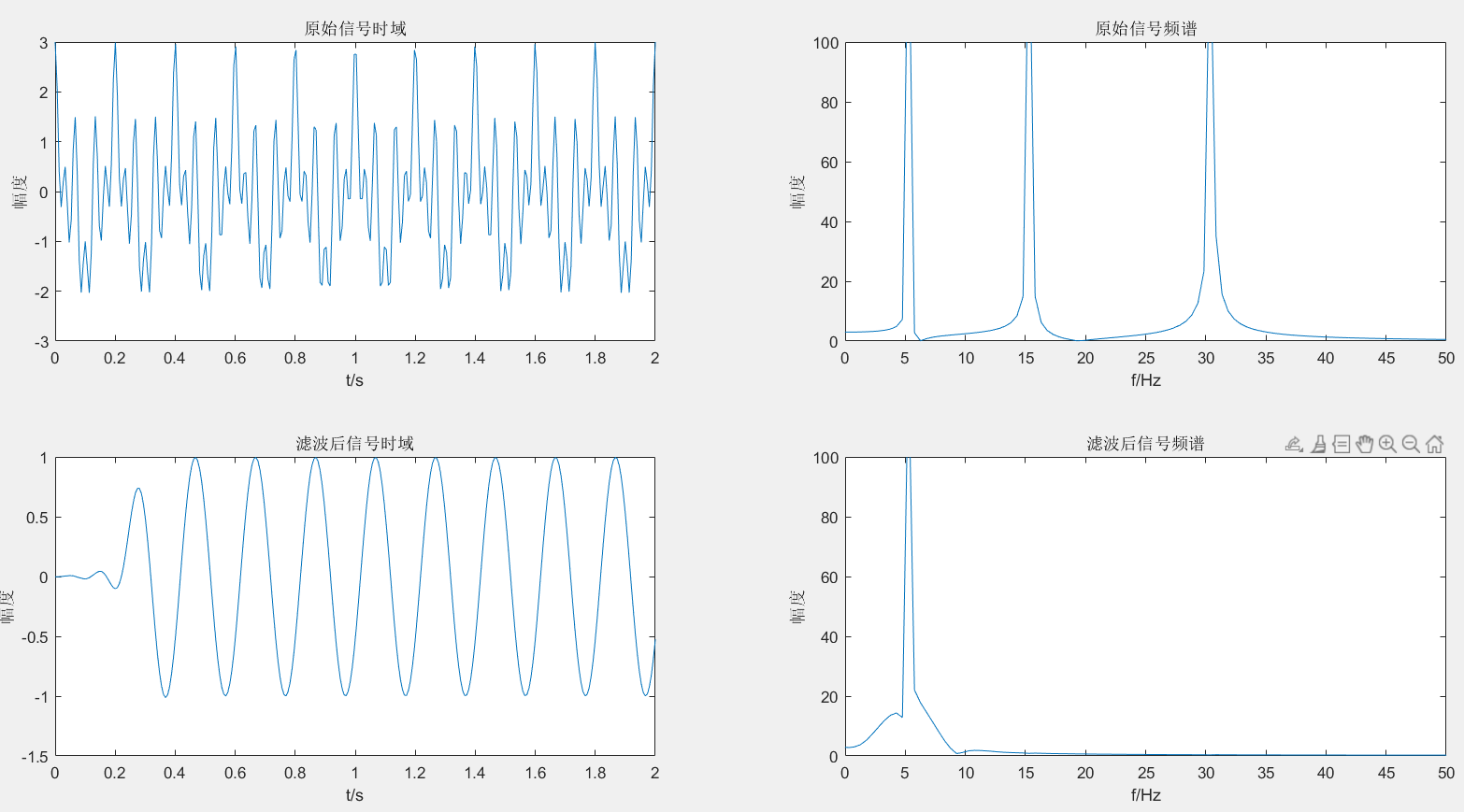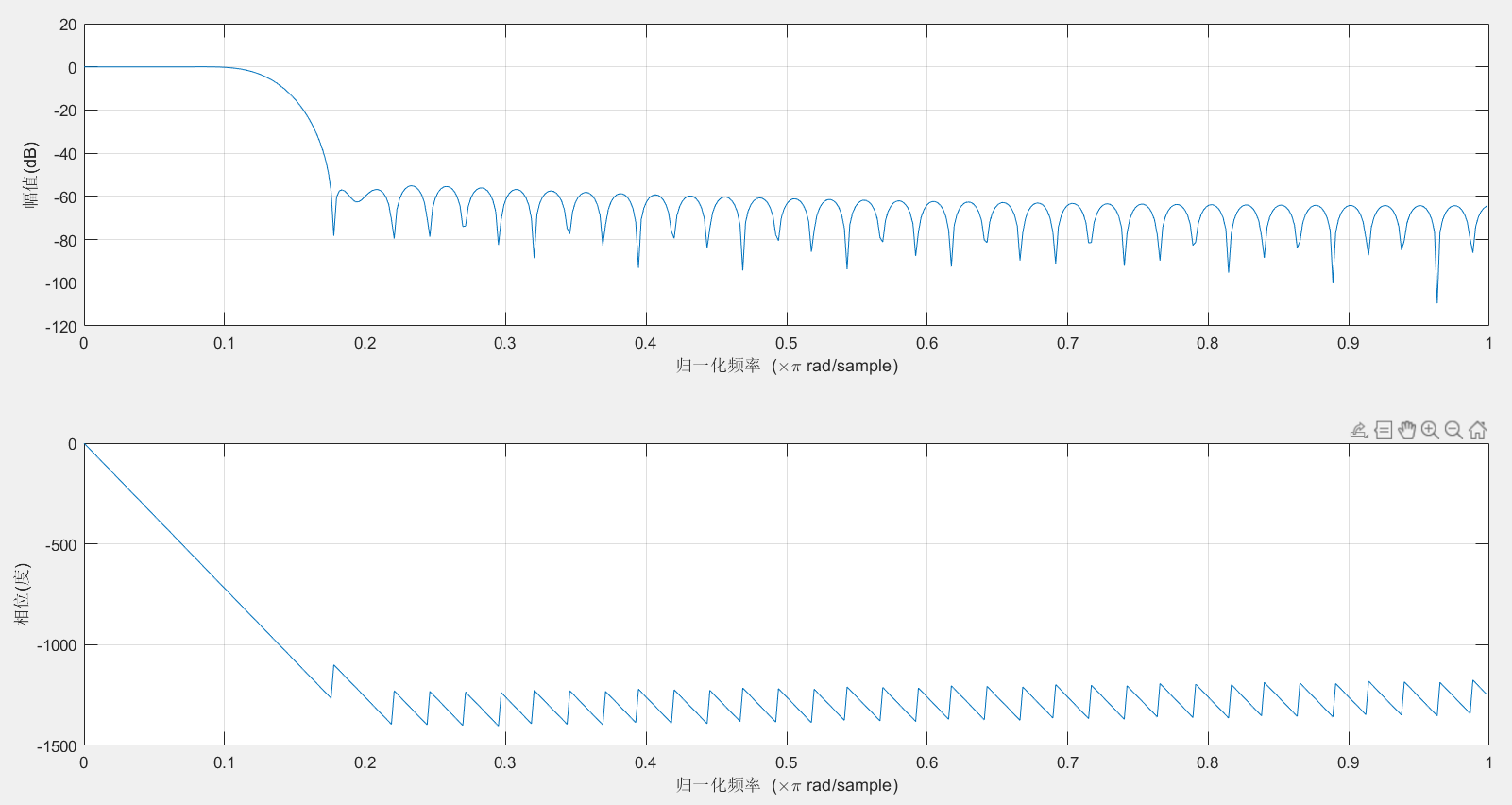二、高通滤波器
1.保留30Hz
clear
clc
f1=5;%第一个点频信号分量频率
f2=15;%第二个点频信号分量频率
f3=30;%第三个点频信号分量频率
fs=150;%采样率
T=2;%时宽
B=25;%FIR截止频率
n=round(T*fs);%采样点个数
t=linspace(0,T,n);
y=cos(2*pi*f1*t)+cos(2*pi*f2*t)+cos(2*pi*f3*t);
figure;
subplot(221)
plot(t,y);
title('原始信号时域');
xlabel('t/s');
ylabel('幅度');
fft_y=fftshift(fft(y));
f=linspace(-fs/2,fs/2,n);
subplot(222)
plot(f,abs(fft_y));
title('原始信号频谱');
xlabel('f/Hz');
ylabel('幅度');
axis([ 0 50 0 100]);
b=fir1(80, B/(fs/2),'high'); %高通
y_after_fir=filter(b,1,y);
subplot(223)
plot(t,y_after_fir);
title('滤波后信号时域');
xlabel('t/s');
ylabel('幅度');
fft_y1=fftshift(fft(y_after_fir));
f=linspace(-fs/2,fs/2,n);
subplot(224)
plot(f,abs(fft_y1));
title('滤波后信号频谱');
xlabel('f/Hz');
ylabel('幅度');
axis([ 0 50 0 100]);
figure;
freqz(b);%数字滤波器频率响应

2.运行结果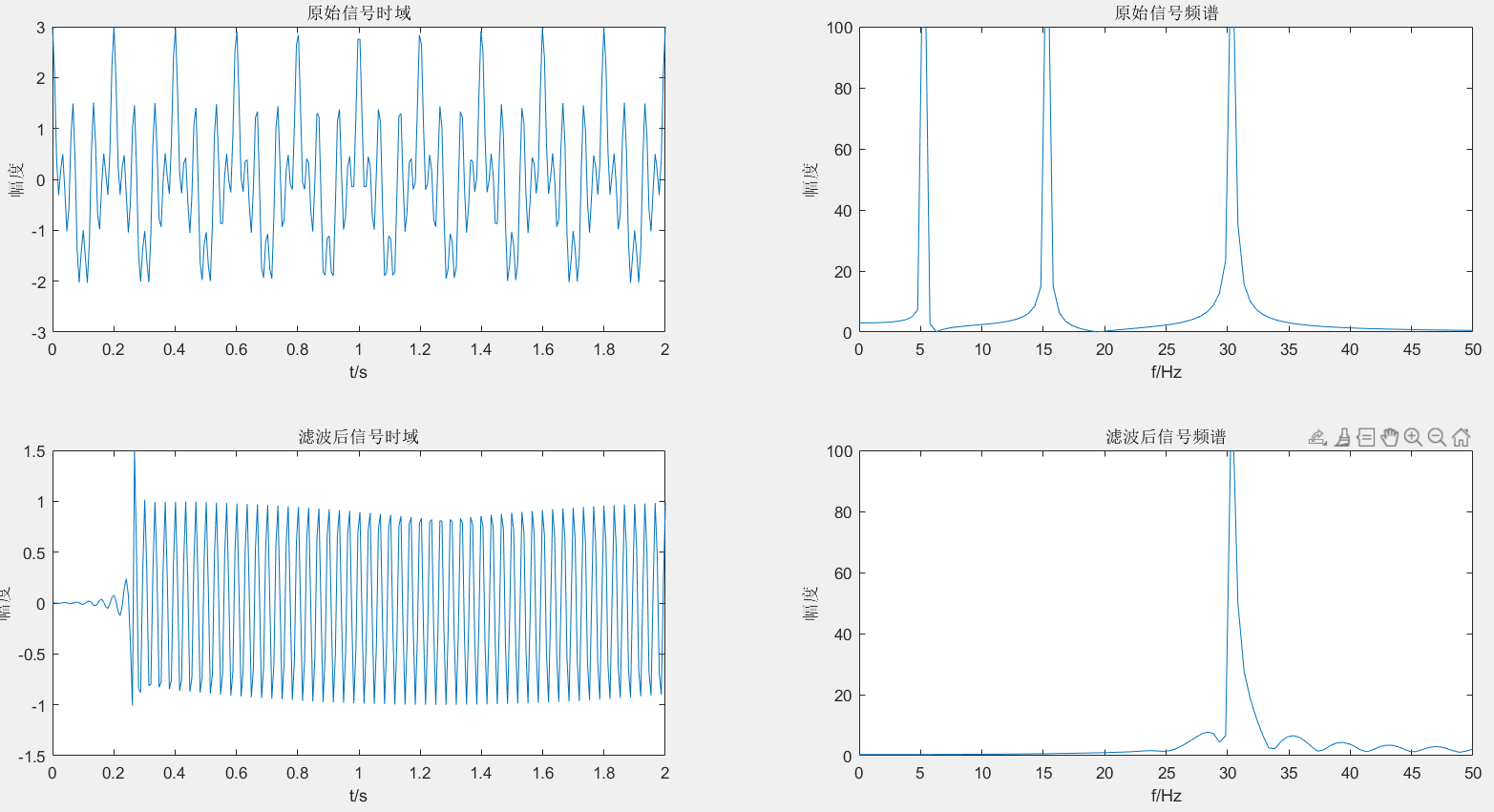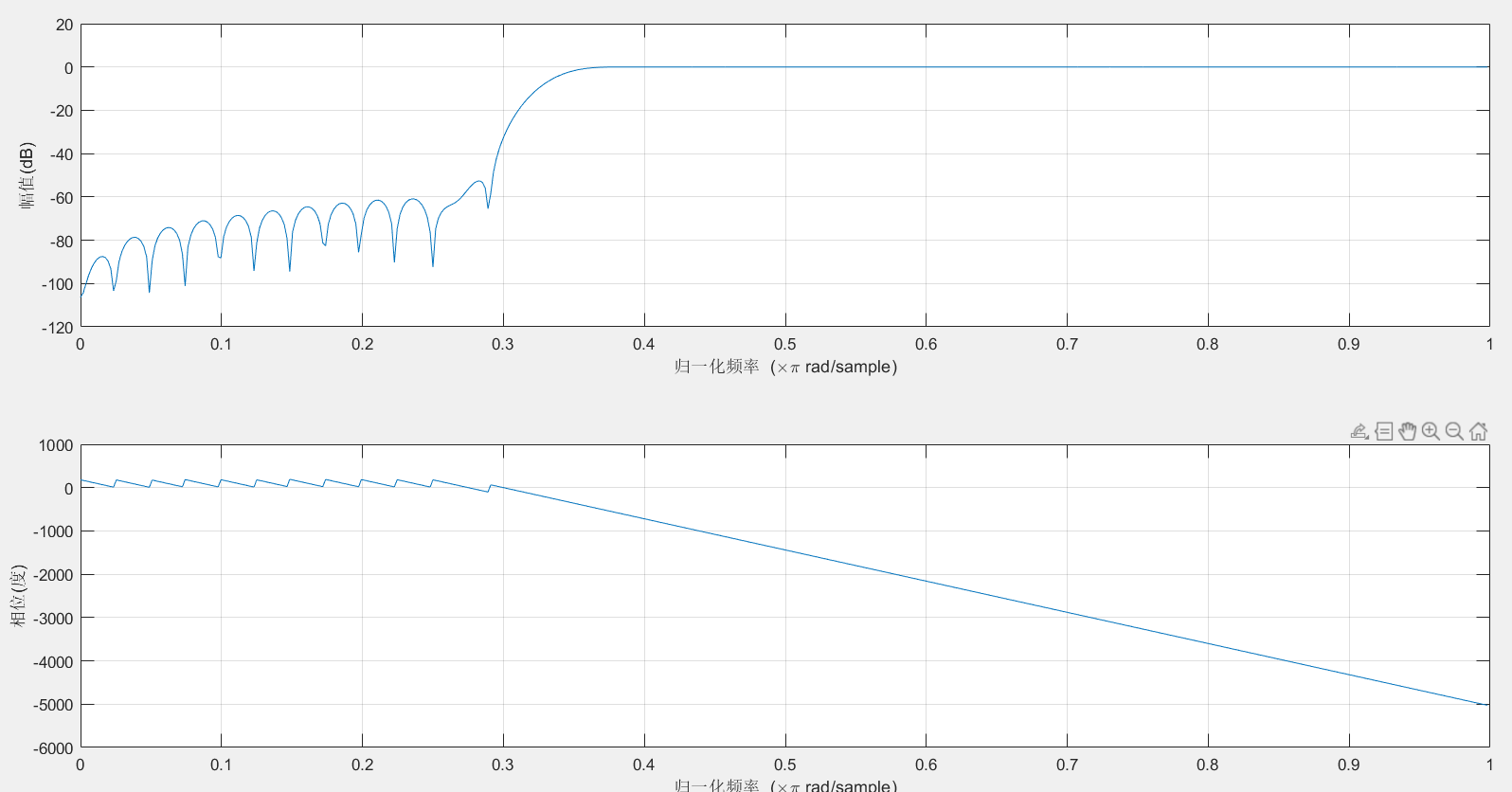三、带通滤波器
1.保留20Hz
clear
clc
f1=5;%第一个点频信号分量频率
f2=15;%第二个点频信号分量频率
f3=30;%第三个点频信号分量频率
fc1=10;
fc2=25;
fs=150;%采样率
[n,Wn,beta,ftype]=kaiserord([7 13 17 23] ,[0 1 0], [0.01 0.01 0.01] ,100) ;
w1=2*fc1/fs; w2=2*fc2/fs ;
window=kaiser(n+1 ,beta) ;%使用kaiser窗函数
b=fir1 (n, [w1 w2],window) ;%使用标准频率响应的加窗设计函数fir1
T=2;%时宽
n=round(T*fs);%采样点个数
t=linspace(0,T,n);
s = cos(2*pi*t*f1) +cos (2*pi*t*f2) +cos (2*pi*t*f3) ;
sf = filter (b,1,s) ;%对信号s进行滤波
f=linspace(-fs/2,fs/2,n);
S=fftshift(fft(s)) ;
SF=fftshift(fft(sf)) ;
figure
subplot(2,2,1) ;
plot(t,s)
%画出时域内的号
subplot(2,2,3) ;
plot(t,sf)
%画出时域内的信号
subplot(2,2,2) ;
f1=abs(S);
f2=abs(SF);
plot(f,f1) ; %画出频域内的信号
title('原始信号频谱');
axis([ 0 50 0 100]);
subplot(2,2,4) ;
plot(f,f2);%画出频域内的信号
title('滤波后信号频谱');
axis([ 0 50 0 100]);
figure;
freqz (b,1,512) ;%数字滤波器频率响应

2.运行结果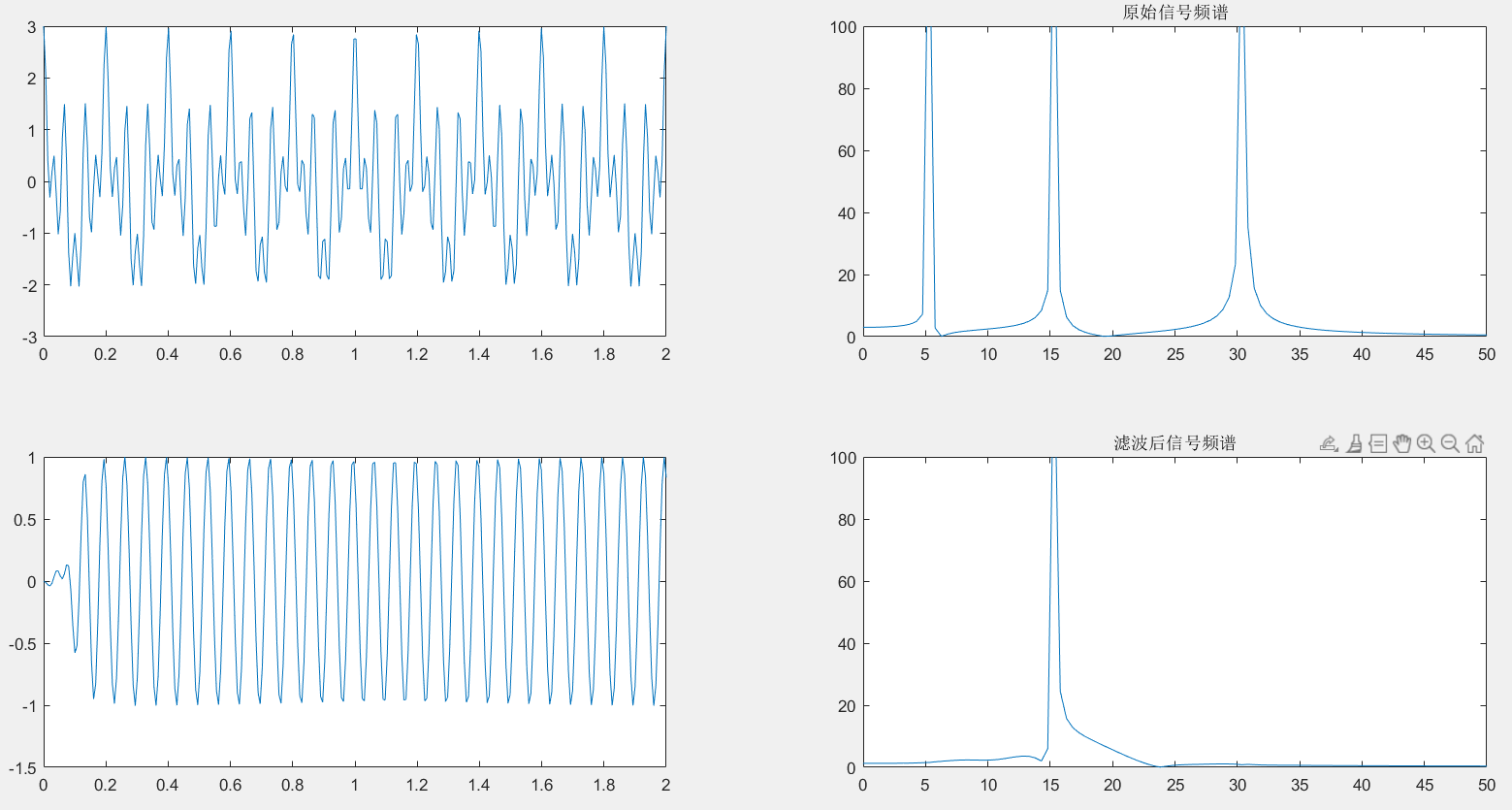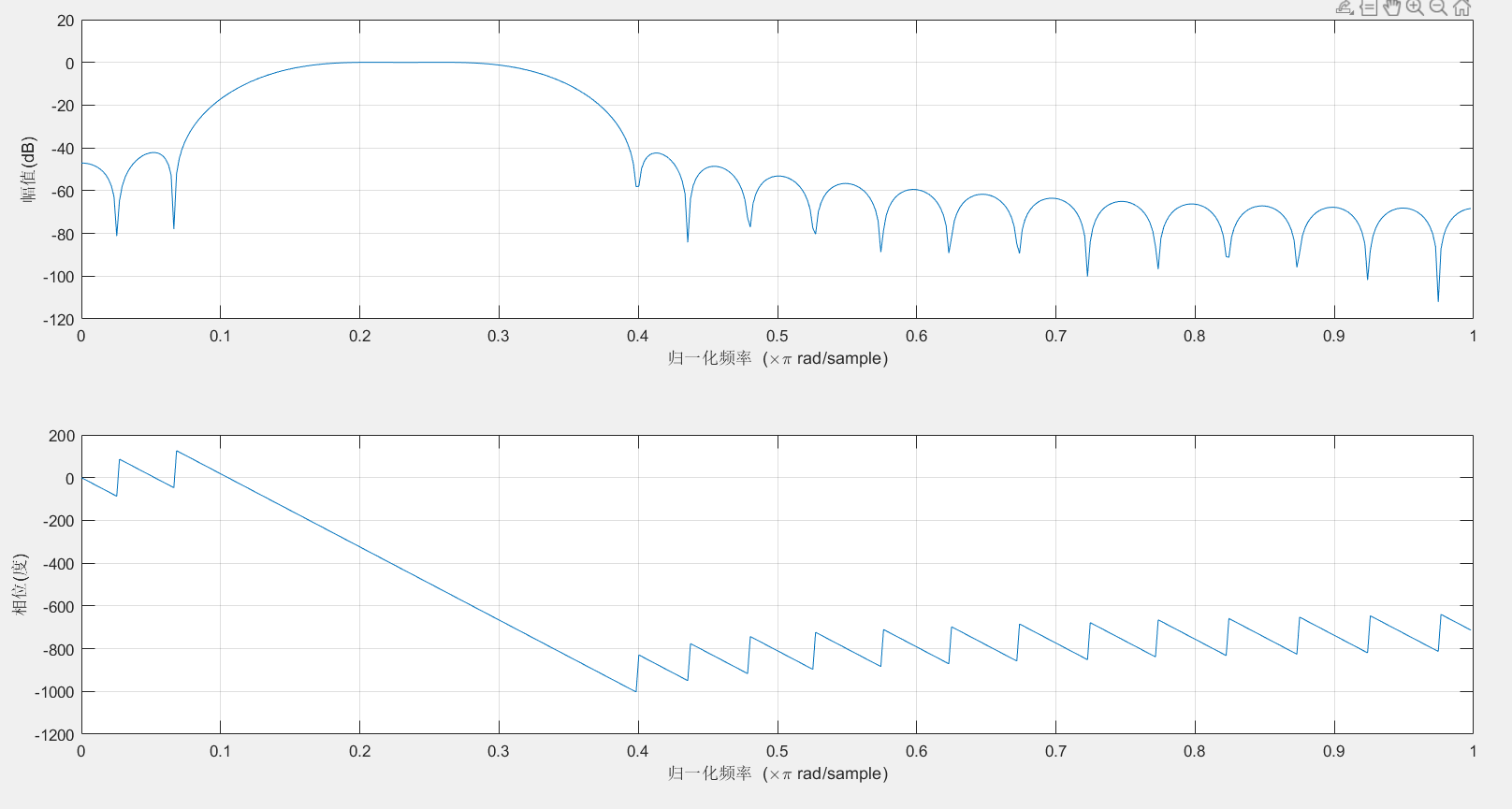四、带阻滤波器
1.滤除5Hz和30Hz
clear
clc
f1=5;%第一个点频信号分量频率
f2=15;%第二个点频信号分量频率
f3=30;%第三个点频信号分量频率
fs=150;
T=2;%时宽
n=round(T*fs);%采样点个数
t=linspace(0,T,n);
y=cos(2*pi*f1*t)+cos(2*pi*f2*t)+cos(2*pi*f3*t);
flp=0;
fhp=30;
fls=15;
fhs=25;
wlp=2*pi*flp/fs;
whp=2*pi* fhp/fs;
wls=2*pi*fls/fs;
whs=2*pi* fhs/fs;
wc=[(wlp+wls)/(2* pi),(whp+whs)/(2*pi)];
delta1=wls-wlp;
delta2=whp-whs;
delta_w=min(delta1 ,delta2);
N=ceil(1.8*pi/delta_w); %不同的窗要选择系数不同
N=N+rem(N,2);
window=boxcar(N+1); %选择窗函数
b=fir1 (28, wc,window) ;
ylb=filter(b,1,y);
f=linspace(-fs/2,fs/2,n);
y1=fftshift(fft(y));
ylb1=fftshift(fft(ylb)) ;
figure(1)
subplot(221)
plot(t,y)
subplot(222)
y1=abs(y1);
plot(f,y1)
title('原始信号频谱');
axis([ 0 50 0 100]);
subplot(223)
plot(t,ylb)
subplot(224)
ylb1=abs(ylb1);
plot(f,ylb1)
title('滤波后信号频谱');
axis([ 0 50 0 100]);
figure
freqz (b,1,512) ;%数字滤波器频率响应


2.运行结果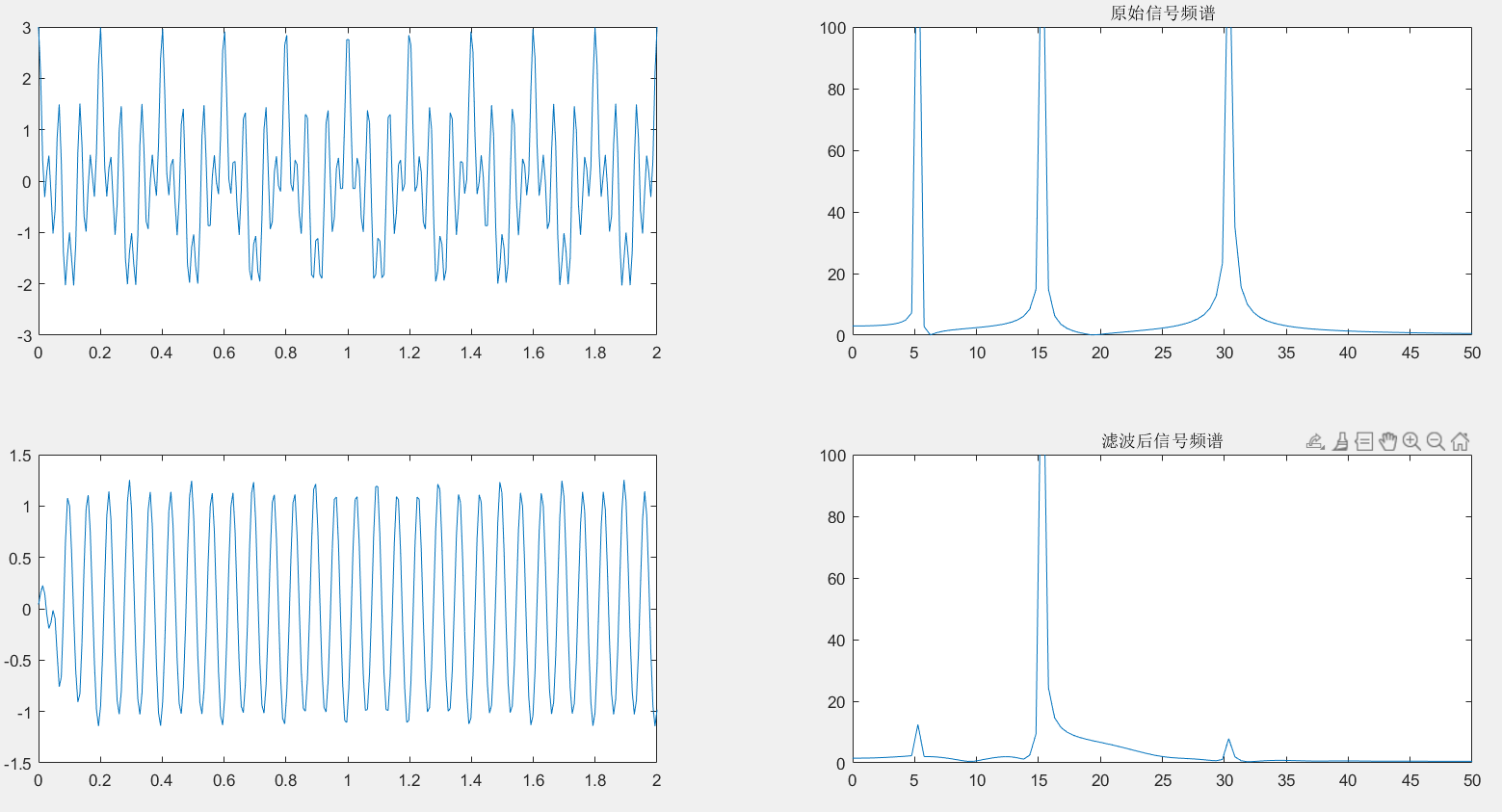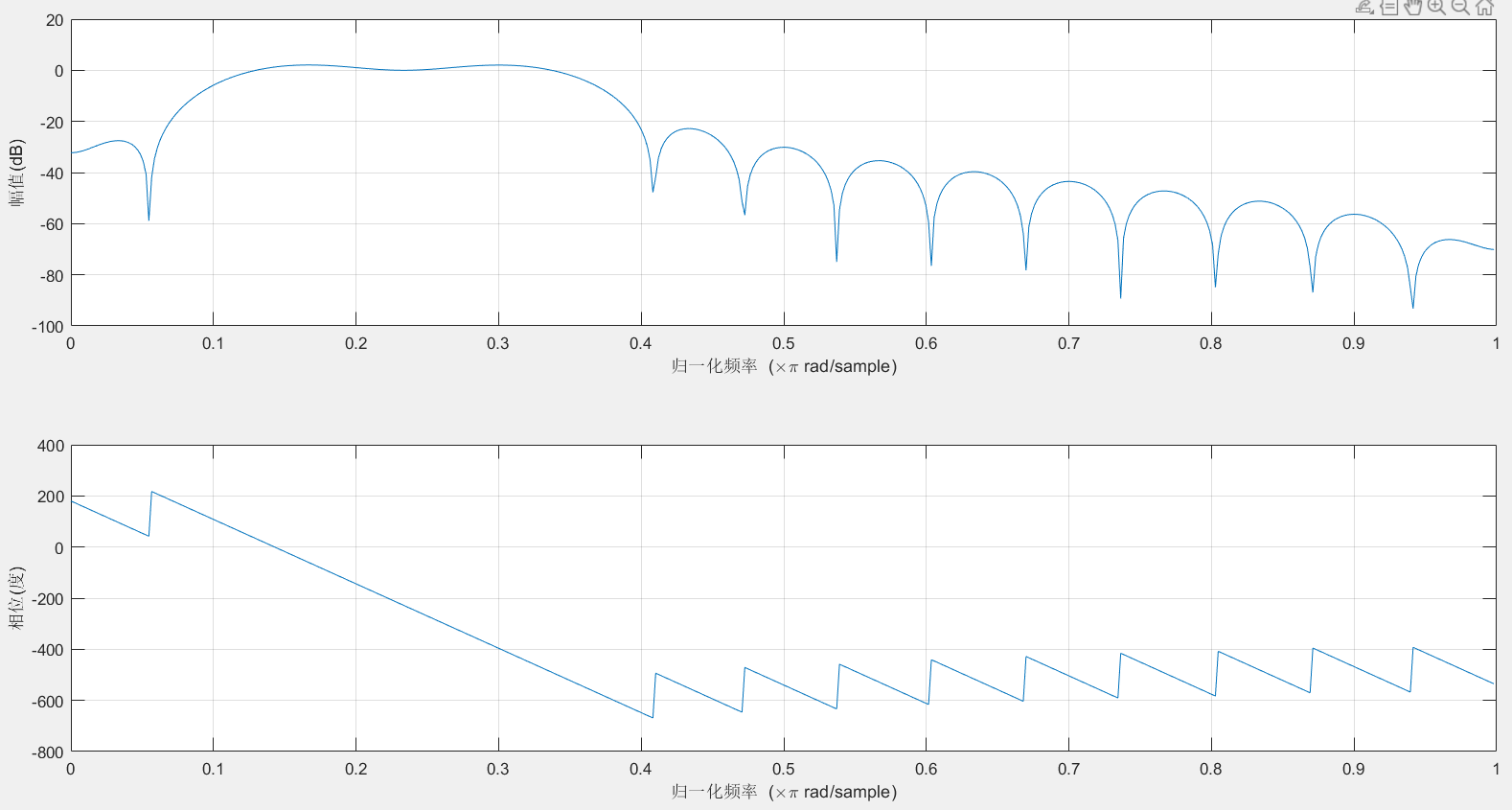最后
不定期发布一些matlab设计内容,敬请期待。包括但不限于如下内容:信号处理、通信仿真、gui设计、matlab appdesigner，simulink仿真。有任何有关MATLAB的问题可加QQ：2802009708进行咨询。或扫码进行添加。展开全文matlab 数字信号处理 filter
• 带阻滤波器matlab代码磁共振成像 这是我在Matlab中执行的静息状态功能MRI时间序列分析，以识别内在的大脑网络 这些代码包括运动校正，图像配准，图像分割，空间归一化，图像平滑，时域滤波，扰动消除，频谱分析，...
• 带阻滤波器matlab代码时域重构与过滤 信息工程小项目2017-18 该项目的主要目的是开发用于设计最佳脉冲响应序列的代码，以对采样数据进行低通滤波，并报告代码及其使用结果。 我使用了多种数值技术，包括优化和积分，...
• 本文提出了一种基于多模一维光子晶体波导（PhCW）的超紧凑型宽带可调谐光学带阻滤波器（OBSF），并对其进行了系统的研究。 对于微型阻带中的波长，PhCW将输入模式耦合到对向传播的高阶模式，然后以锥形辐射，从而在...研究论文
• 目录题目分析步骤3. 结果4. 程序 题目        设计一个带阻滤波器，滤除受到1000Hz正弦噪声...1000HZ正弦噪声的频谱图是一根(对称两根)谱线,带阻滤波器的阻带覆盖该谱线,然后...
目录1. 题目2.分析3.步骤4.	结果5.	程序
1. 题目
设计一个带阻滤波器，滤除受到1000Hz正弦噪声污染的音频信号。加入正弦噪声，幅度0.1，频率1000Hz。
2.分析
1000HZ正弦噪声的频谱图是一根(对称两根)谱线,带阻滤波器的阻带覆盖该谱线,然后变换为时域形成一个序列,将该序列与音频信号序列进行卷积即可滤去1000HZ正弦噪声,但也会滤去原信号1000HZ左右频率信号。
使用切比雪夫1型滤波器来设计滤波器，重点是阻带通带频率的调整。然后使用切比雪夫1型滤波器获得的两个向量参数对音频信号进行卷积滤波，使用移动平均滤波器来对含噪数据进行平滑处理。此示例使用 filter 函数计算沿数据向量的平均值。移动平均值滤波器沿数据移动长度为 windowSize 的窗口，并计算每个窗口中包含的数据的平均值。以下差分方程定义向量 x 的移动平均值滤波器：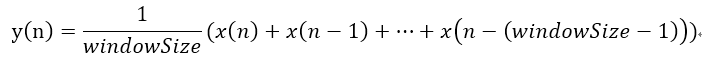3.步骤
1.读取音频文件，格式为wav:
[audio,fs]=audioread(‘audio.wav’);
%取一个通道的信号
audio = audio(:,1); %双通道变单通道
2.产生一个正弦信号A=0.1，f=1000Hz:
noise=0.1sin(10002pi/fstt’);%加噪声 1000HZ
%tt’是(1:n)’，有公式 $\omega = 2\pi f/{f_s}$计算
3.选取合适的技术参数，设计滤波器，得到滤波系数[b，a]
选择带阻切比雪1型滤波器，
通带截止频率:Fp=[306 337]; %通带边界频率
通带衰减: rp=1dB
阻带截止频率:Fs=[316 327]; %阻带边界频率
阻带衰减: rs=50dB；
对应角频率(模拟滤波器的边界频率):
wp = 2*pi*Fp/Ft;
ws = 2*pi*Fs/Ft ;   %求出待设计的模拟滤波器的边界频率
切比雪阶数:
[n,wn]=cheb1ord(wp,ws,rp,rs); %滤波器的最小阶数为n，wn为系统频带
滤波参数计算:
[bz,az]=cheby1(n,rp,wp,‘stop’);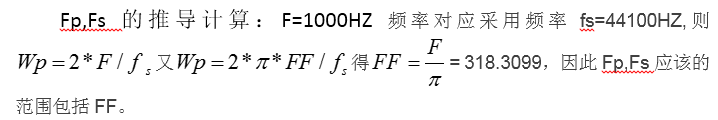4.进行滤波
z=filter(bz,az,s);
4.	结果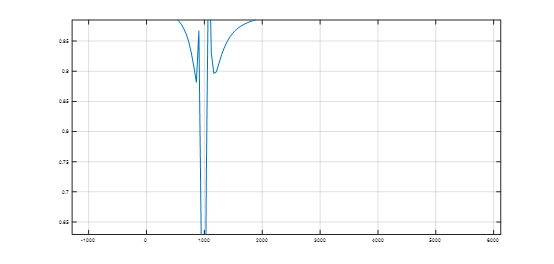带阻滤波器频谱图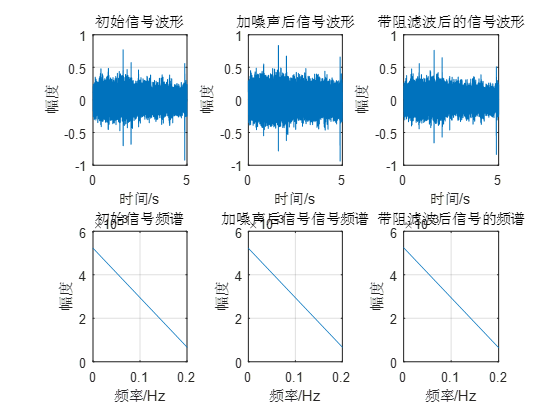原始信号、加噪信号、带阻滤波信号比较图
5.	程序
clear
clc
[audio,fs]=audioread('audio.wav');%声音读取
audio = audio(:,1); %双通道变单通道
n=length(audio);

T = 1/fs;%采样间隔
t = (0:n-1)*T;%时间轴
f = (0:n-1)/n*fs;%频率轴

%快速傅里叶变换
audio_fft=fft(audio,n)*T;

%加噪声
tt =(1:n);
noise=0.1*sin(1000*2*pi/fs*tt');%加噪声 1000HZ
s=audio+noise;
s_fft=fft(s,n)*T;

%设计IIR低通滤波器
rp = 1;  %通带最大衰减1db
rs=50;  %阻带最小衰减50db
Ft=fs;
Fp=[306 336]; %通带边界频率
Fs=[316 326]; %阻带边界频率
% Fp=4000; %通带边界频率
% Fs=5000; %阻带边界频率
wp = 2*pi*Fp/Ft;
ws = 2*pi*Fs/Ft ;   %求出待设计的模拟滤波器的边界频率
% [N,wn]=buttord(wp,ws,rp,rs,'s');    %低通滤波器的阶数和截止频率
% [b,a]=butter(N,wn,'s');             %S域频率响应的参数即：滤波器的传输函数
% [bz,az]=bilinear(b,a,0.5);          %利用双线性变换实现频率响应S域到Z域的变换
[n,wn]=cheb1ord(wp,ws,rp,rs); %滤波器的最小阶数为n，wn为系统频带
[bz,az]=cheby1(n,rp,wp,'stop');
figure(2);%带阻滤波器特性
[h,w]=freqz(bz,az);
title('IIR带阻滤波器');
plot(w*fs/(2*pi),abs(h));  %确保1000HZ落在截断处
grid;

%滤波
z=filter(bz,az,s);
z_fft=fft(z)*T;     %滤波后的信号频谱
figure(1);
%绘出原始音频时域波
subplot(2,3,1);
plot(t,audio);
xlabel('时间/s');
ylabel('幅度');
title('初始信号波形');
grid;
%绘出原始音频频域频谱

subplot(2,3,4);
audiof = abs(audio_fft);
plot(f(1:(n-1)/2),audiof(1:(n-1)/2));
title('初始信号频谱');
xlabel('频率/Hz');
ylabel('幅度');
grid;
%绘出加噪音频时域波
subplot(2,3,2)
plot(t,s);
title('加噪声后信号波形')
xlabel('时间/s');
ylabel('幅度');
grid;
%绘出加噪音频频域频谱
subplot(2,3,5)
sf = abs(s_fft);
plot(f(1:(n-1)/2),sf(1:(n-1)/2));
xlabel('频率/Hz');
ylabel('幅度');
title('加噪声后信号信号频谱');
grid;
%绘出滤波音频时域波
subplot(2,3,3);
plot(t,z);
title('带阻滤波后的信号波形');
xlabel('时间/s');
ylabel('幅度');
grid;
%绘出滤波音频频域波
subplot(2,3,6);
zf = abs(z_fft);
plot(f(1:(n-1)/2),zf(1:(n-1)/2));
title('带阻滤波后信号的频谱');
xlabel('频率/Hz');
ylabel('幅度');
grid;
audio_final = [audio;s;z];%原始语音，加噪语音，滤波语音的合成音频矩阵
sound(audio_final,fs); %播放语音




展开全文• 窗函数法设计线性相位 FIR 数字带阻滤波器需要以下步骤: ①选择窗函数w(n)，计算窗函数长度N ② 构造希望逼近的频率响应函数Hd(ejω) ③ 计算hd(n) ④加窗 (2)附参数表 （3）设计过程 ①选择窗函数w(n)，计算窗函数...
ωs 为0.3pi和0.4pi，ωp 为0.2pi和0.5pi，ap为1dB,as为46dB,请按窗函数法设计 FIR 滤波器的步骤，设计该滤波器，详细分析设计过程
（数字信号期末试题，如有错误欢迎指正）
（7月6日更 附matlab）
(1)分析
窗函数法设计线性相位 FIR 数字带阻滤波器需要以下步骤:
①选择窗函数w(n)，计算窗函数长度N
② 构造希望逼近的频率响应函数Hd(ejω)
③ 计算hd(n)
④加窗
(2)附参数表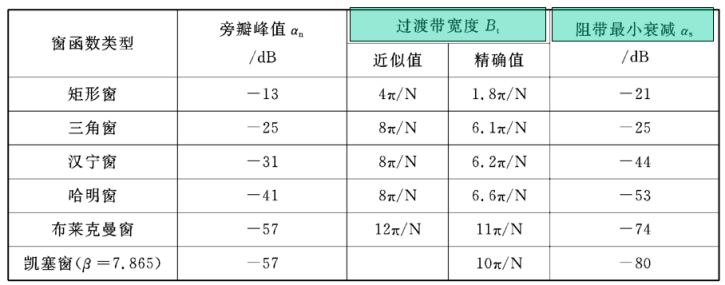（3）设计过程
①选择窗函数w(n)，计算窗函数长度N
已知阻带最小衰减as=46dB，查表选择哈明窗
本题过渡带宽度Bt≤ωlp－ωls=0.1π
哈明窗的精确过渡带宽度Bt=6.6π/N
所以要求Bt=6.6π/N≤π/0.1，解得N≥66。
N须取奇数，取N=67
查阅得出哈明窗的w(n)，代入数值得：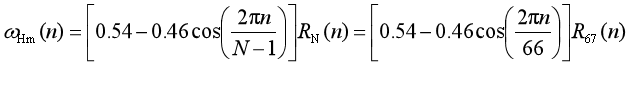②构造希望逼近的频率响应函数Hd(e^jw)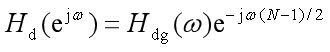选用理性相性带阻滤波器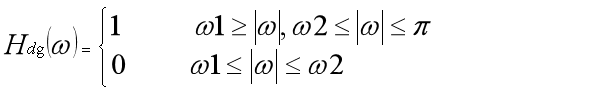所以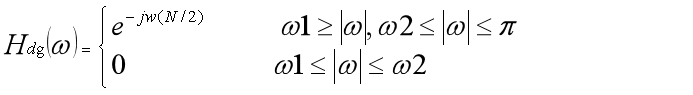本题的理想线性相位带阻滤波器的上下截止频率为：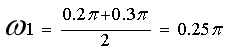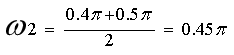③ 计算hd(n)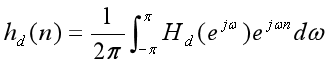计算出hd(n)：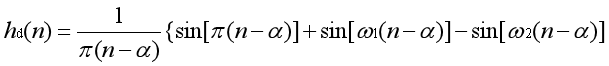其中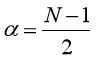代入数据得33
所以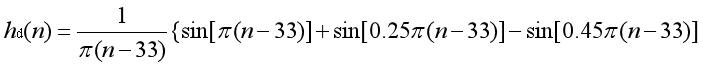④加窗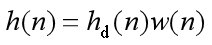将已算出数据代入得：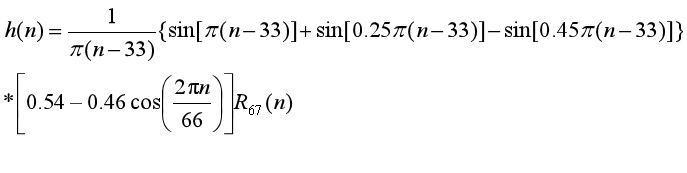(4)matlab
wlp=0.2*pi;%下通带截止频率
wls=0.3*pi;%阻带下限频率
wus=0.4*pi;%阻带上限频率
wup=0.5*pi;%上通带截止频率
wc=[(wlp+wls)/2/pi,(wus+wup)/2/pi];%截止频率在通带和阻带边界频率的中点
B=wls-wlp;%迁移域
N=ceil(6.6*pi/B)-1;%正向取整数，根据过渡带宽等于窗函数主瓣宽求窗函数的最小长度
n=0:N-1;
alpha=(N-1)/2;%求滤波器的相位延迟
m=n-alpha+eps;%eps为MATLAB系统的精度
%hd=sin(wc*m)/(m*pi);%求理想滤波器脉冲响应  %维度不同，无法运行
window=hamming(N);
[h1,w]=freqz(window,1);
figure(1);
subplot(2,1,1)
stem(window,'.');
xlabel('n');
title('哈明窗函数');
subplot(2,1,2)
plot(w/pi,20*log(abs(h1)/abs(h1)))
grid on;
xlabel('w/pi');
ylabel('幅度（dB）');
title('哈明窗函数频谱');
hn=fir1(N-1,wc,'stop');
[h2,w]=freqz(hn,1,512);
figure(2);
subplot(2,1,1)
stem(n,hn,'.');
xlabel('n');
ylabel('h(n)');
title('单位脉冲响应');
subplot(2,1,2)
plot(w/pi,20*log(abs(h2)/abs(h2(1))));
grid on;
xlabel('w/pi');
ylabel('幅度（dB）');
title('哈明窗带阻滤波器的幅度特性');

Ts=0.001;
fs=1/Ts;
x=cos(2*pi*25*n*Ts)+cos(2*pi*35*n*Ts)+cos(2*pi*45*n*Ts);
y=x.*window';%加窗函数进行滤波处理
xfft=fft(x,N);
xfft=xfft.*conj(xfft)/N;
y1=fft(y,N);
y2=y1.*conj(y1)/N;
figure(3);%滤除前后的信号对比。
subplot(2,2,1);plot(n,x);axis([0 60 -4 4]);grid;
xlabel('时间 (s)');ylabel('幅度');title('输入信号');
subplot(2,2,3);plot(n,y);axis([0 60 -4 4]);grid;
xlabel('时间 (s)');ylabel('幅度');title('滤波器输出');
subplot(2,2,2);plot(n*fs/N,xfft);axis([0 fs/2 min(xfft) max(xfft)]);grid;
xlabel('频率 (Hz)');ylabel('Magnitude (dB)');title('输入信号');
subplot(2,2,4);plot(n*fs/N,y2);axis([0 fs/2 min(y2) max(y2)]);grid;
xlabel('频率 (Hz)');ylabel('Magnitude (dB)');title('滤波器输出');

运行结果：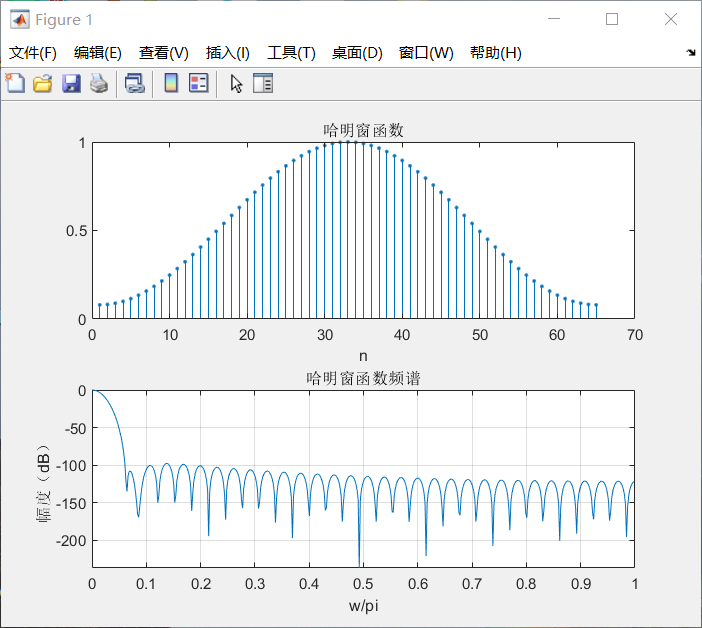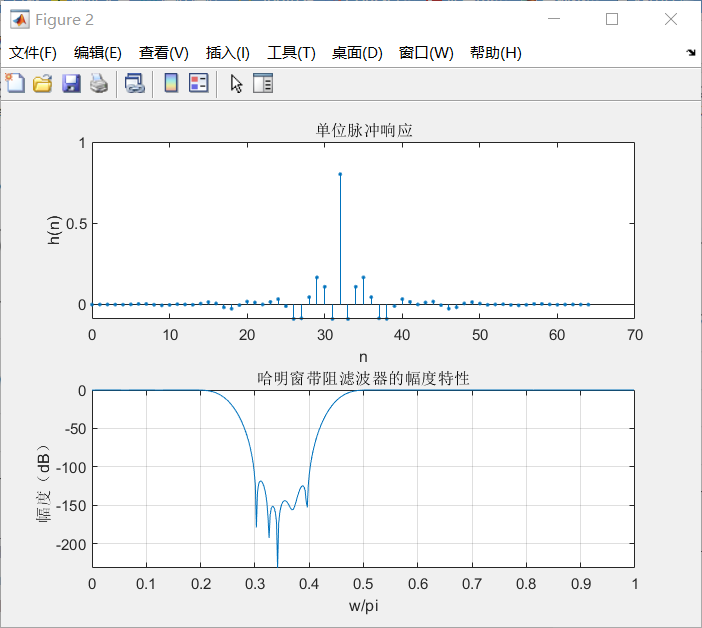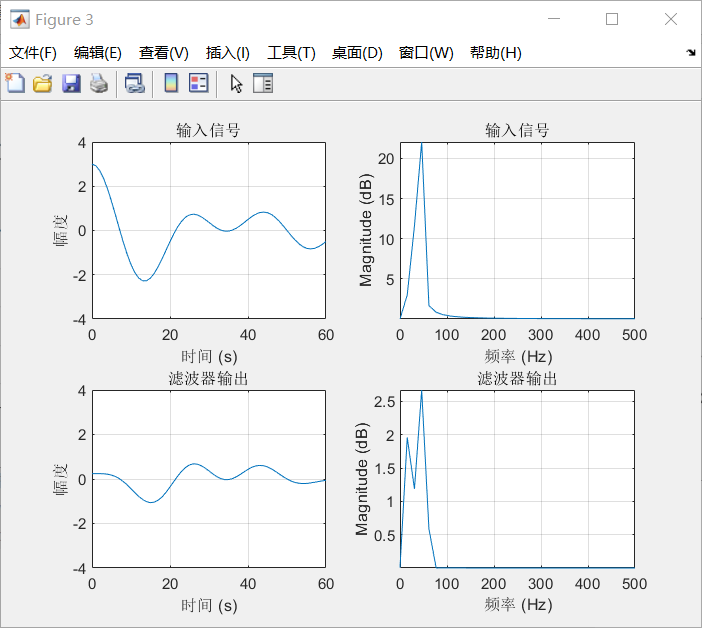对滤出后信号频域分析可以发现：本滤波器完成带阻滤波器功能


展开全文数字信号处理
• 本文利用阻抗分析法对连续变化的微带滤波器的传输特性进行研究，分析设计了连续变化的带阻和低通微带滤波器，给出了滤波器的形状和结构尺寸。实际制作并测试了几种典型的微带滤波器，理论结果与测试结果比较一致。
• ## 低通滤波器分析

千次阅读 2021-01-13 13:51:25
滤波器的分类：低通滤波器（LPF）、高通滤波器(HPF)、带通滤波器（BPF）、带阻滤波器（BEF）。下边介绍的滤波器均为无源的。 1、无源低通滤波器 允许信号中较低频率成分通过滤波器时，这种滤波器叫做低通滤波器。 ...
• 2.1 IIR滤波器与FIR滤波器分析比较 2.2 FIR滤波器的原理 3 FIR滤波器的仿真步骤 二、源代码 %-------------------------------------------------------------------------- %利用kaiser窗设计低通...
• 1.自己产生一个序列，序列频带能完全分开，做FFT谱分析。 2.设计低通，高通，带通，带阻四种数字滤波器，来滤掉波形。
• 摘要： 提出了一种谐振腔间通过电容耦合和基于LTCC技术的集总带通滤波器（带通滤波器是指能通过某一频率范围内的频率分量、但将其他范围的频率分量衰减到极低水平的滤波器，与带阻滤波器的概念相对）结构。...
• 滤波器 你 欧拉公式 阻抗 百度百科对阻抗做如下定义：在具有电阻、电感和电容的电路里，对电路中的电流所起的阻碍作用叫做阻抗。阻抗常用Z表示，是一个复数，实部称为电阻，虚部...带阻滤波器 全通滤波器（APF） ...信号处理
• 摘要： 提出了一种谐振腔间通过电容耦合和基于LTCC技术的集总带通滤波器（带通滤波器是指能通过某一频率范围内的频率分量、但将其他范围的频率分量衰减到极低水平的滤波器，与带阻滤波器的概念相对）结构。...
• 介绍了信号与系统基础知识、常用信号变换、离散系统结构、IIR数字滤波器设计、FIR数字...IIR滤波器设计包括模拟和数字低通、高通、带通与带阻滤波器设计，以及基于冲激响应不变法和双线性Z变换法的IlR滤波器设计等；
• 要求 估算例 1的带阻滤波器在中心频率10kHz处的衰减量。可使用的电感Q值为100，假定电容器无损耗，为了保持理论通带特性，确定Q是否足够？ 　解 ①计算所有调谐电路谐振时的等效阻抗。   　②在10kHz处的等效...
• 滤波器按照频带又可分为低通、高通、带通和带阻四种，其中高通、带通和带阻滤波器均可由低通滤波器由频率变换得到，本文仅考虑低通滤波器的分析与设计。N阶巴特沃斯滤波器由于在0频率处的2N-1阶导数都等于零，所以......